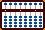###Multiplying Negative Numbers

When multiplying and dividing negative numbers, I wanted to see if I could create a technique whereby I could calculate using only the positive numbers and still come up with the correct negative answer. This is what I've come up with. Using this technique, the only time you'll have to work with the negative complement is;

i)  If your problem begins with a negative number, it'll need to be placed correctly on the soroban.
ii) If the answer is a negative, obviously you'll need to be able to read it.

The reason I like this is, I can do all the work using actual positive numbers while the negative complementary numbers underneath work themselves out naturally of their own accord. I find the technique particularly beneficial if I have to multiply larger numbers such as  -58 *37 = -2146  (see Example 2 below), or when doing a long string of calculations that might include a combination of something like: (98 - 140)6  ?7  +78 = 42.

The following technique has its little quirks and although it does seem to work, I can't explain why. (See Gary Flom's Explanation)

The method works best using a modern 1:4 bead soroban and will use two Japanese soroban techniques:

Remember to follow Kojima's rules for reading negative numbers. In order to see the true complement, 1 is always added to the right-hand most rod that shows value.

In the following examples the parenthesized (**) numbers are the positive numbers actually displayed on the soroban. For example if  -12 were the negative complementary number, (88) would be the number displayed.

Example 1:  5 -12 *8 = -56

Step 1: Place 5 on the soroban

Step 2: Subtract 12 to equal  -7(3).

Step 3: Now  -7(3) *8 ==> Here's the trick. Rather than multiplying  -7 *8, multiply (3) *8  to equal (24) instead. The negative complementary number at this point is  -76 while the number displayed on the soroban is (24).

Step 4: ***Here's the quirk*** Now you must subtract the multiplier from the **first number in the product**. In this example 8 is the multiplier, so subtract 8 from the 2 on rod B. This is not possible, so solve it by adding 1 to rod A and then subtracting 8 from rods AB. (see below).

A B C
2 4
-8   ==> ??? (can't be done)

Add 1 to A and subtract 8 from AB [or, if you prefer, 80 from BC].

A B C
1 2 4
- 8
=   4 4

With the numbers on the soroban showing (44), the resulting complementary number will read  -56, which is the answer.

Example 2:  -58 *37 = -2146

Step 1: Set  - 58(42) on the soroban and the multiplier 37 somewhere on the left.

Step2: Now  -58(42) *37 ==> Rather than multiplying  -58 *37, multiply (42) *37  instead. Using Kojima's method for multiplication, the soroban shows (1554) while the negative complementary answer reads 8446.

Step 3: Once again as in the first example subtract the multiplier. In this case subtract 37, from the **first two numbers of the product** (see below).

A B C D E
1 5 5 4
-3 7     ==> ??? (can't be done)

Again, so solve it by adding 1 to A and subtracting 37 from 115 on rods BCD (or if you prefer, 3700 from BCDE)

A B C D E
1 1 5 5 4
-3 7
=   7 8 5 4

With the numbers on the soroban showing (7854) the resulting complementary number will read  -2146, which is the answer.

As you can see the real advantage here is this. In the problem above we could have multiplied 58 *37 to equal 2146. But then we'd then have to turn around and set the negative answer -2146 (7854) onto the soroban using the negative complementary number approach. This would be time consuming because it adds another step to the process. Using this method the correct numbers are set naturally of their own accord. From here if we needed to continue to add, subtract or multiply further numbers it's easily done because  -2146 is already correctly set on the soroban.

Members of the Yahoo soroban group offer some important insights into my method;

Here's a link to an exercise on how to change back and forth between Celsius to Fahrenheit with an emphasis on when they have negative values.

Print Page (.pdf format,112kb)